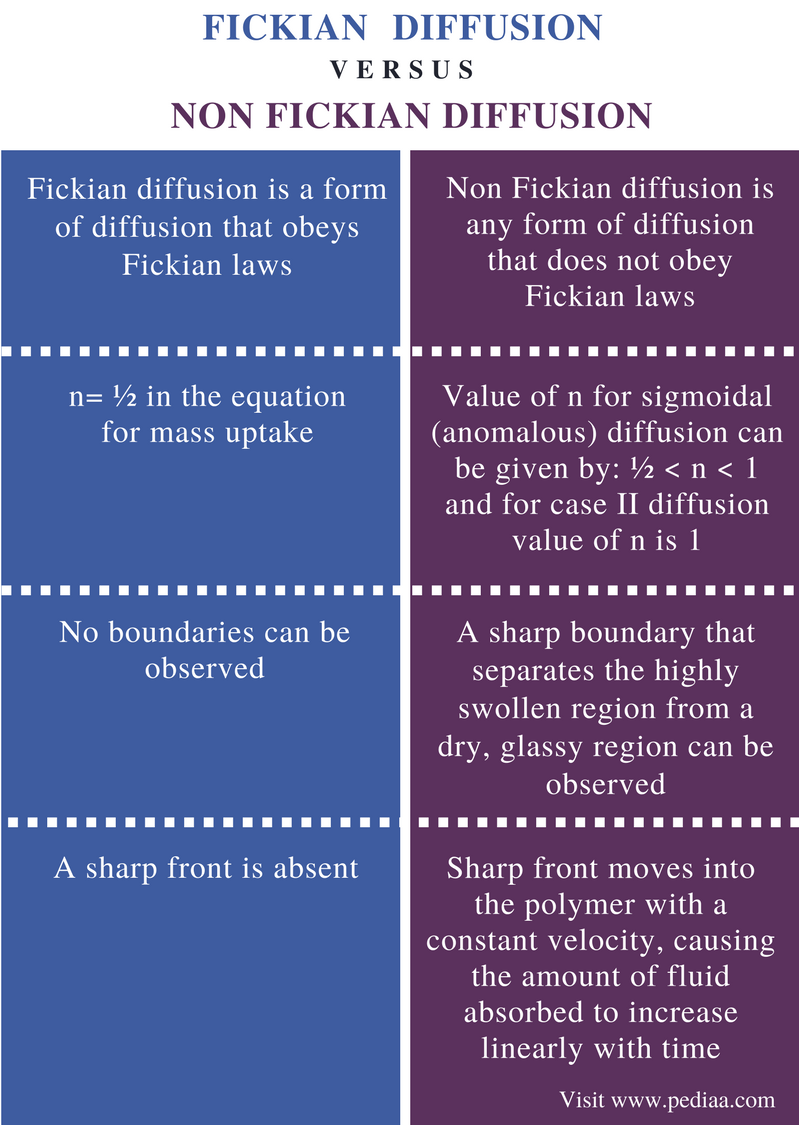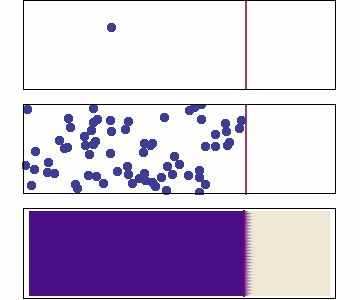# Difference Between Fickian and Non Fickian Diffusion

## Main Difference – Fickian vs Non Fickian Diffusion

Diffusion is the movement of molecules from a region of high concentration to a region of low concentration. In other words, the molecules move through a concentration gradient. Therefore, the factors that affect the concentration gradient will also affect the diffusion. However, the diffusion that takes place in solid substances such as polymers is very different from that of liquids and gases. Fick’s laws are a set of equations that can be used to explain the diffusion in solids. Fickian and Non Fickian Diffusion are two forms of diffusion that are described using Fick’s laws. Fickian diffusion obeys the Fickian laws whereas non Fickian diffusion does not obey the Fickian laws. The main difference between Fickian and Non Fickian Diffusion is the presence or absence of boundaries; there are no boundaries in Fickian diffusion whereas non Fickian diffusion has a sharp boundary separating the highly swollen region from a dry, glassy region.

### Key Areas Covered

1. What are Fick’s Laws
– Explanation, First Law, Second Law
2. What is Fickian Diffusion
– Definition, Explanation
3. What is Non Fickian Diffusion
– Definition, Different Types
4. What is the Difference Between Fickian and Non Fickian Diffusion
– Comparison of Key Differences

Key Terms: Diffusion, Diffusivity, Fickian Diffusion, Fick’s First Law, Fick’s Second Law, Non Fickian Diffusion, Stress, Swelling## What are Fick’s Laws

Fick’s Laws of diffusion were introduced by Adolf Fick in 1855. These laws give the simplest description of diffusion. There are two Fick’s laws as given below.

1. The molar flux through a unit area of the material is proportional to the concentration gradient.
2. The concentration changes as a function of time to the change in flux with respect to position.

### Fick’s First Law

According to Fick’s first law, the molar flux (flow of solute) through a unit area of the material is proportional to the concentration gradient. Here, the proportionality constant is known as diffusion coefficient. Diffusion is the movement of components from a high concentration to a low concentration across a concentration gradient. This is the simple idea of the Fick’s first law. The law can be given in a mathematical equation as;

J   =   -D (dϕ/dx)

J is the diffusion flux; its dimension is the amount of substance per unit area per unit time; hence the unit is mol m−2 s−1.

D is the diffusion coefficient. It is also known as the diffusivity. The dimension of this component is area per unit time; hence the unit is m2/s.

Φ is the concentration. It is given by the unit mol/m3.

x is the position of a solute. The dimension for this component is length. It is given by unit m.

Diffusion coefficient is proportional to the following factors.

• Squared Velocity Of The Diffusing Particles
• Temperature
• Viscosity Of The Fluid
• Size Of Particles

### Fick’s Second Law

Fick’s second law of diffusion is used to predict how the concentration changes with time when diffusion occurs. According to Fick’s second law, the concentration changes as a function of time to the change in flux with respect to position. It is given by a partial differentiation equation as below.

δϕ/δt   = D  δ2ϕ/δx2

ϕ is the concentration (a dimension that depends on time and location (x)).

t is time (given by s)

D is the diffusion coefficient.

X is the position (given by dimensions of length).

The above equation is given for diffusion in one dimension. For two or more dimensions, more complex equations are given.

## What is Fickian Diffusion

In gases and liquids, diffusion does not create a stress field. But in solids, a particular stress is created due to the presence of swelling penetrants. This stress can cause even cracks, which in other words, morphological changes are induced. Then these swelling and stress fields can affect the diffusion. The effects of swelling and stresses can be:

• through morphological changes
• through solubility changes
• through changing diffusivity (it depends on stresses)
• through stress dependence of the flux.

A fundamental criterion of Fickian diffusion is that the surface concentration attains its equilibrium value immediately upon a change in conditions and remains constant through the sorption process. For example, in a resin matrix system, the polymer chain segments at the surface must instantaneously reach saturation.

Fickian diffusion is rarely observed for the transport of a liquid through a glassy polymer. If the mass uptake M can initially be represented by,

M = ktn

t is the time, and k and n are constants for Fickian diffusion, n= ½.

## What is Non Fickian Diffusion

Non Fickian diffusion is the diffusion that occurs without obeying the Fick’s laws of diffusion. In 1946, the concept of the non Fickian diffusion was brought forward during the discussion held on swelling and shrinking by Faraday Society. This concept states that in some polymer systems, sharp boundaries that move linearly with time exist between the swollen and un-swollen regions. After about 20 years, Alfrey named this as “Case II diffusion” which is now recognized as a type non Fickian diffusion. There are four types of non Fickian diffusion as given below.

1. Classical diffusion
2. Sigmoidal diffusion
3. Case II diffusion
4. Two step diffusion

If the mass uptake M can initially be represented by,

M = ktn

t is the time, and k and n are constants, then the followings can be predicted.

• Value of n for sigmoidal (anomalous) diffusion can be given by: ½ < n < 1.
• Value of n for case II diffusion is 1.Figure 1: Molecular Diffusion

### Features of Non Fickian Diffusion

• A sharp boundary that separates the highly swollen region from a dry, glassy region
• The sharp front moves into the polymer with a constant velocity, causing the amount of fluid absorbed to increase linearly with time
• A small Fickian precursor exists in the dry region ahead of the front
• There is an initial induction time, during which the sharp boundary is established near the film surface.

## Difference Between Fickian and Non Fickian Diffusion

### Definition

Fickian Diffusion: Fickian diffusion is a form of diffusion that obeys the Fickian laws.

Non Fickian Diffusion: Non Fickian diffusion is any form of diffusion that does not obey the Fickian laws.

### Value of n in Mass Uptake Equation

Fickian Diffusion: For Fickian diffusion, n= ½ in the equation for mass uptake.

Non Fickian Diffusion: Value of n for sigmoidal (anomalous) diffusion can be given by: ½ < n < 1 and for case II diffusion value of n is 1.

### Presence of Boundaries

Fickian Diffusion: No boundaries can be observed in Fickian diffusion.

Non Fickian Diffusion: A sharp boundary that separates the highly swollen region from a dry, glassy region can be observed in non Fickian diffusion.

### Movement of Sharp Front

Fickian Diffusion: A sharp front is absent in Fickian diffusion.

Non Fickian Diffusion: The sharp front  in non Fickian diffusion moves into the polymer with a constant velocity, causing the amount of fluid absorbed to increase linearly with time

### Conclusion

Fickian and non Fickian diffusion are two forms of diffusion. Fickian diffusion can be explained using Fick’s law, but not non Fickian diffusion. The main difference between Fickian and Non Fickian Diffusion is that Fickian diffusion obeys the Fickian laws whereas non Fickian diffusion does not obey the Fickian laws.

##### Reference:

1. “Viscoelastic (Non Fickian) Diffusion.” The Canadian Journal of Chemical Engineering, vol. 83, Dec. 2005, pp. 913–915., Available here.
2. “Multiphysics Cyclopedia.” COMSOL, Available here.
3. “Fick’s laws of diffusion.” Wikipedia, Wikimedia Foundation, 22 Jan. 2018, Available here.

##### Image Courtesy:

1. “DiffusionMicroMacro” By Sbyrnes321 – Own work (Public Domain) via Commons Wikimedia